# Chapter 18 Areas of Circle, Sector & Segment RS Aggarwal Solutions MCQ Class 10 Maths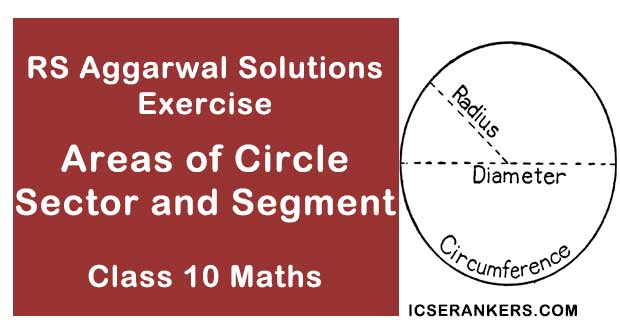Chapter Name RS Aggarwal Chapter 18 Areas of Circle, Sector & Segment Book Name RS Aggarwal Mathematics for Class 10 Other Exercises Exercise 18AExercise 18B Related Study NCERT Solutions for Class 10 Maths

### Areas of Circle, Sector & Segment MCQ Solutions

1. The area of a circle is 38.5 cm2. The circumference of the circle is

A. 6.2 cm

B. 12.1 cm

C. 11 cm

D. 22 cm

Solution

Let the radius if the circle be r

Given, Area of circle = 38.5 cm2

Area of circle = πr2

⇒ πr2 = 38.5

Since π = 22/7

⇒ 22/7 × r2 = 38.5

⇒ r2 = 38.5 × (7/22)

⇒ r2 = 12.25

⇒ r = √12.25 = 3.5 cm

∴ Radius of circle = 3.5 cm

Circumference of circle = 2πr

= 2 × 22/7 × 3.5 cm

= 22 cm

∴ Circumference of the circle is 22 cm

Let the radius if circle be r

Given, Area of circle = 38.5 cm2

Area of circle = πr2

πr2 = 38.5

Since = 22/7

∴ πr2 = 38.5

⇒ 22/7 × r2 = 38.5

⇒ r2 = 12.25

⇒ r = √12.25 = 3.50 cm

∴ Radius of circle = 3.5 cm

Circumference of circle = 2πr

= 2 × 22/7 × 3.5

= 22 cm

∴ Circumference of the circle is 22 cm.

2. The area of a circle is 49π cm2. Its circumference is

A. 7π cm

B. 14π cm

C. 21π cm

D. 28π cm

Solution

Let the radius if circle be r

Given, Area of circle = 49π cm2

Area of circle = πr2

πr2 = 49π

Since = 22/7

∴ πr2 = 49π

⇒ r2 = 49

⇒ r = √49 = 7 cm

∴ Radius of circle = 7 cm

Circumference of circle = 2πr

= 2 × r × 7 cm

= 14π cm

∴ Circumference of the circle is 14π cm.

3. The difference between the circumference and radius of a circle is 37 cm. The area of the circle is

A. 111 cm2

B. 84 cm2

C. 154 cm2

D. 259 cm2

Solution

Let the radius if circle be r

Circumference of circle = 2πr

Difference between the circumference and radius of a circle = 37 cm

⇒ 2πr – r = 37 cm

⇒ 2 × 22/7 × r – r = 37 cm

⇒ 44/7 × r – r = 37 cm

⇒ (44/7 – 1) × r = 37 cm

⇒ 37/7 × r = 37 cm

⇒ r = 37 × 7/37

⇒ r = 7 cm

Area of circle = πr2

= 22/7 × 7 × 7 cm2

= 22/7 × 49 cm2

= 22 × 7 cm2

= 154 cm2

∴ Area of the circle is 154 cm2

4. The perimeter of a circular field is 242 m. The area of the field is

A. 9317 m2

B. 18634 m2

C. 4658 m2

D. none of these

Solution

Let the radius if circular field be r

Perimeter of circular field = 2πr

Perimeter of circular field = 242 m

⇒ 2πr = 242 m

⇒ 2 × 22/7 × r = 242 m

⇒ r = 242 × 1/2 × 7/22 = 38.5 m

∴ Radius of circular field = 38.5 m

Area of the filed = πr2

= 22/7 × 38.52 m2

= 22/7 × 1482.5 m2 = 4658.5 m2

∴ Area of the field = 4658.5 m2

5. On increasing the diameter of a circle by 40%, its area will be increased by

A. 40%

B. 80%

C. 96%

D. 82%

Solution

Let the radius if circle be r

Area of circle = A = πr2

So, new radius r’ = r + 40/100 × r = 1.4r

New Area of circle = A’ = πr’2 = π × (1.4r)2

= 1.96πr2

Percentage increase in area = (A’ – A)/A × 100

= (1.96π r2 – πr2)/πr2 × 100

= 0.96 × 100

= 96

∴ Increase in area = 96%

6. On decreasing the radius of a circle by 30%, its area is decreased by

A. 30%

B. 60%

C. 45%

D. none of these

Solution

Let the radius if circle be r

Area of circle = A = πr2

So, New Radius r’ = r – 30/100 × r = 0.7 r

New Area of circle = A’ = πr’2 = π × (0.7r)2

= 0.49 πr2

Percentage decrease in area = (A – A’)/A × 100

= (πr2 - 0.49πr2)/πr2 × 100

= 0.51 × 100

= 51

∴ Decrease in area = 51%

7. The area of a square is the same as the area of a circle. Their perimeters are in the ratio

A. 1 : 1

B. 2 : π

C. π : 2

D. √π : 2

Solution

Let the length of the side of the square be a

Let the radius if circle be r

Area of a square = a2

Area of circle = πr2

Area of a square = Area of a circle

a2 = πr2

⇒ a = √π × r

Perimeter of circle = 2πr

Perimeter of square = 4a

= 4√πr

Perimeter of square)/(Perimeter of circle) = 4√πr/2πr

Perimeter of circle = √π/2

Ratio of perimeter of circle and square = √π: 2

8. The circumference of a circle is equal to the sum of the circumferences of two circles having diameters 36 cm and 20 cm. The radius of the new circle is

A. 16 cm

B. 28 cm

C. 42 cm

D. 56 cm

Solution

Let the bigger circle be C1 and other circles be C2 and C3

Radius of circle C1 = r1

Diameter of circle C2 = 36 cm

Radius of circle C2 = r2 = 36/2 cm = 18 cm

Diameters of circle C3 = 20 cm

Radius of circle C3 = r3 = 20/2 cm = 10 cm

Circumference of circle C2 = 2πr2

= 2 × π × 18 cm

= 36π cm

Circumference of circle C3 = 2πr3

= 2 × π × 10 cm

= 20π cm

Circumference of circle C1 = Circumference of circle C2 + Circumference of circle C3

⇒ 2πr1 = 2πr2 + 2πr3

⇒ 2πr1 = 36π + 20π

⇒ 2πr1 = 56π

⇒ r1 = 28 cm

Radius of circle C1 = r1 = 28 cm

9. The area of a circle is equal to the sum of the areas of two circles of radii 24 cm and 7 cm. The diameter of the new circle is

A. 25 cm

B. 31 cm

C. 50 cm

D. 62 cm

Solution

Let the bigger circle be C1 and other circles be C2 and C3

Radius of circles C1 = r1

Radius of circle C2 = r2 = 24 cm

Radius of circle C3 = r3 = 7 cm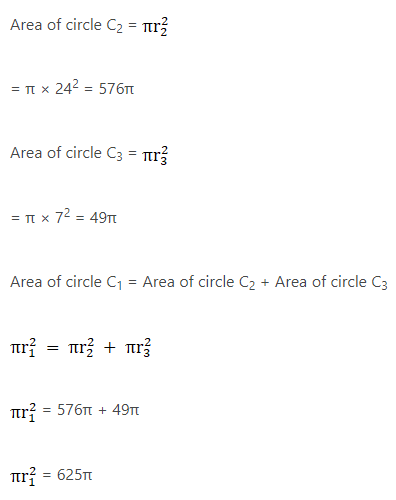r1 = 25 cm

Diameter of new circle = 25 × 2 cm

= 50 cm

10. If the perimeter of square is equal to the circumference of a circle then the ratio of their areas is

A. 4 : π

B. π: 4

C. π : 7

D. 7 : π

Solution

Let the length of the side of the square be a

Let the radius if circle be r

Perimeter of circle = 2πr

Perimeter of square = 4a

Perimeter of circle = Perimeter of square

⇒ 2πr = 4a

a = π × r/2

Area of square = a2

Area of circle = πr2

Area of square)/(Area of circle) = a2/πr2

(Area of square)/(Area of circle) = (πr/4)2/πr2

(Area of square)(Area of circle) = π2r2/4πr2

Area of square)/Area of circle|) = π/4

Ratio of area of square to circle = π : 4

11. If the sum of the areas of two circles with radii R1 and R2 is equal to the area of a circle of radius R then

A. R1 + R2 = R

B. R1 + R2 < R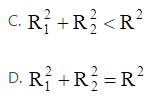Solution

Let the three circles be C1, C2 and C

Area of circle C = Area of circle C1 + Area of circle C2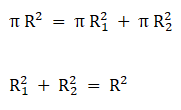12. If the sum of the circumferences of two circles with radii R1 and R2 is equal to the circumference of a circle of radius R then

A. R1 + R2 = R

B. R1 + R2 > R

C. R1 + R2 < R

D. None of these

Solution

Let three circles be C1, C2 and C

Circumference of circle C = Circumference of circle C1 + Circumference of circle C2

⇒ 2πR = 2πR1 + 2πR2

R = R1 + R2

13. If the circumference of a circle and the perimeter of a square are equal then

A. area of the circle = area of the square

B. (area of the circle) > (area of the square)

C. (area of the circle) < (area of the square)

D. None of these

Solution

Let the length of the side of the square be a

Let the radius if circle be r

Perimeter of circle = 2πr

Perimeter of square = 4a

Perimeter of circle = Perimeter of square

2πr = 4a

⇒ a = π × r/2

Area of square = a2

= (π × r/2)2

= π/4 × πr2

Area of circle = πr2

Seeing the co-efficient of πr2

1 > π/4

∴ πr2 > π/4 × πr2

So, (area of the circle) > (area of the square)

14. The radii of two concentric circles are 19 cm and 16 cm respectively. The area of the ring enclosed by these circles is

A. 320 cm2

B. 330 cm2

C. 332 cm2

D. 340 cm2

SolutionRadius of circle 1 = r1 = 19 cm

Radius of circle 2 = r2 = 16 cm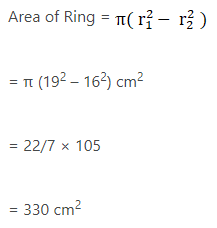15. The areas of two concentric circles are 1386 cm2 and 962.5 cm2. The width of the ring is

A. 2.8 cm

B. 3.5 cm

C. 4.2 cm

D. 3.8 cm

Solution

Let the radius of circle 1 & 2 be R1 and R2 respectively

Area of circle 1 = 1386 cm2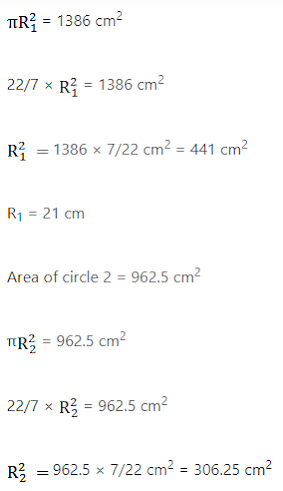R2 = 17.5 cm

Width of the ring = R1 – R2

= 21 – 17.5

= 3.5 cm

16. The circumferences of two circles are in the ratio 3 : 4. The ratio of their areas is

A. 3 : 4

B. 4 : 3

C. 9 : 16

D. 16 : 9

Solution

Circumference of circle C1 = 2πr1

Circumference of circle C2 = 2πr2

(Circumference of circle C1)/(Circumference of circle C2) = 2πr1/2πr2 = 3/4

r1/r2 = 3/4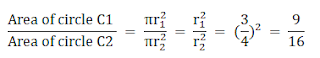∴ Ratio of two circles = 9 : 16

17. The areas of two circles are in the ratio 9 : 4. The ratio of their circumferences is

A. 3 : 2

B. 4 : 9

C. 2 : 3

D. 81 : 16

Solution

r1/r2 = 3/2

(Circumference of circle C1)/(Circumference of circle C2) = 2πr1/2πr2 = r1/r2 = 3/2

Ratio of their circumferences = 3 : 2

18. The radius of a wheel is 0.25 m. How many revolutions will it making in covering 11 km?

A. 2800

B. 4000

C. 5500

D. 7000

Solution

Radius of wheel = r = 0.25 m

Distance the wheel travels = 11 km = 11000 m

In 1 revolution wheel travels 2πr distance

No. of revolutions a wheel makes = (distance travelled by the wheel)/2πr

= 11000/(2π × 0.25)

= 11000/(2 × 22/7 × 0.25)

= (11000 × 7)/(2 × 22 × 0.25

= 7000 revolutions

19. The diameter of a wheel is 40 cm. How many revolutions will it make in covering 176 cm?

A. 140

B. 150

C. 160

D. 166

Solution

Diameter of wheel = 40 cm

Radius of wheel = r = 40/2 cm = 20 cm

Distance the wheel travels = 176 m = 17600 cm

In 1 revolution wheel travels 2πr distance

No. of revolutions a wheel makes = (distance travelled by the wheel)/2πr

= 17600/(2π × 20)

= 17600/(2 × 22/7 × 20)

= 17600 × 7)/(2 × 22 × 20)

= 140 revolutions

20. In making 1000 revolutions, a wheel covers 88 km. The diameter of the wheel is

A. 14 m

B. 24 m

C. 28 m

D. 40 m

Solution

Distance the wheels travels = 88 km = 88000 m

In 1 revolution wheel travels 2πr distance

No. of revolutions a wheel makes = (distance travelled by the wheel)/2πr

No. of revolutions a wheel makes = 1000

r = (distance travelled by the wheel)/(2π × No. of revolutions a wheel makes) = 88000/(2 × 22/7 × 1000)

= (88000 × 7)/(2 × 22 × 1000)

r = 14 m

Radius of wheel = 14 m

Diameter of wheel = 2 × 14 m = 28 m

21. The area of a sector of angle θ° of a circle with radius R is

A. 2πR2θ/180

B. 2πR2θ/360

C. πR2θ/180

D. πR2θ/360

Solution

Area of a sector of angle θ° of a circle with radius R = area of circle × θ/360

= πR2θ/360

22. The length of an arc of a sector of angle θ° of a circle with radius R is

A. 2πRθ /180

B. 2πRθ/360

C. πR2θ/180

D. πR2θ/360

Solution

Length of an arc of a sector of angle θ° of a circle with radius R

= Circumference of circle × θ/360

= 2πRθ/360

23. The length of the minute hand of a clock is 21 cm. The area swept by the minute hand in 10 minutes is

A. 231 cm2

B.210 cm2

C .126 cm2

D. 252 cm2

Solution

Length of the minute hand of a clock = 21 cm

∴ Radius = R = 21 cm

In 1 minute, minute hand sweeps 6°

So, in 10 minutes, minute hand will sweep 10 × 6° = 60°

Area swept by minute hand in 10 minutes = Area of a sector of angle of a circle with radius R = πR2θ/360

= 22/7 × 21 × 21 × 60/360

= 231 cm2

24. A chord of a circle of radius 10 cm subtends a right angle at the centre. The area of the minor segments (given, π = 3.14) is

A. 32.5 cm2

B. 34.5 cm2

C. 28.5 cm2

D. 30.5 cm2

Solution

Radius of circle = R = 10 cm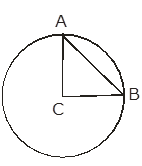Area of minor segment = Area of sector subtending 90° - Area of triangle ABC

Area of sector subtending 90° = πR2θ/ 360

= 3.14 × 10 × 10 × 90/360 cm2

= 78.5 cm2

Area of triangle ABC = 1/2 × AC × BC

= 1/2 × 10 × 10 cm2

= 50 cm2

Area of Minor segment = 78.5 cm2 – 50 cm2

= 28.5 cm2

25. In a circle of radius 21 cm, an arc subtends an angle of 60° at the centre. The length of the arc is

A. 21 cm

B. 22 cm

C. 18.16 cm

D. 23.5 cm

Solution

Radius of circle = R = 21 cm

Angle subtended by the arc = 60°

Length of an arc of a sector of angle θ° of a circle with radius R = 2πRθ/360

Length of arc = 2 × 22/7 × 21 × 60/360 cm

= 22 cm

26. In a circle of radius 14 cm, an arc subtends an angle of 120° at the centre. If √3 = 1.73 then the area of the segment of the circle is

A. 120.56 cm2

B. 124.63 cm2

C. 118.24 cm2

D. 130.57 cm2

Solution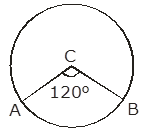Radius of Circle = R = 14 cm

Angle subtended by the arc = θ = 120°

Area of sector subtending 120° = πR2θ/360

= 22/7 × 14 × 14 × 120/360 cm2

= 205.33 cm2

In triangle ABC

AC = BC = 14 cm = R

Area of triangle ABC = 1/2 × base × height

= 2 × 1/2 × R sin θ/2 × R × cos θ/2

= 2 × 1/2 × 14 × 14 × sin 60° × cos 60°

= 84.77 cm2

Area of segment = Area of sector subtending 120° - Area of triangle ABC

= 205.33 – 84.77 cm2

= 120.56 cm2

### Areas of Circle, Sector & Segment

1. In the given figure, a square OABC has been inscribed in the quadrant OPBQ. If OA = 20 cm then the area of the shaded region is [take π = 3.14]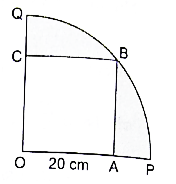A. 214 cm2

B. 228 cm2

C. 242 cm2

D. 248 cm2

Solution

Length of side of square = OA = 20 cm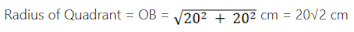Radius of Quadrant = πR2 × θ/360 = 3.14 × 202 × 20√2 × 90/360

= 628 cm2

Area of square = a2 = 202 cm2 = 400 cm2

Area of Shaded region = Area of Quadrant – Area of Square

= 628 cm2 – 400 cm2

= 228 cm2

2. The diameter of a wheel is 84 cm. How many revolutions will it make to cover 792 m?

A. 200

B. 250

C. 300

D. 350

Solution

Diameter of wheel = 84 cm

Radius of wheel = r = 84/2 cm = 42 cm

Distance the wheel travels 792 m = 79200 cm

In 1 revolution wheel travels 2πr distance

No. of revolutions a wheel makes = (distance travelled by the wheel)/2πr

= 79200/(2π × 42)

= 79200/(2 × 22/7 × 42)

= (79200 × 7)/(2 × 22 × 42)

= 300 revolutions

3. The area of a sector of a circle with radius r, making an angle of x° at the centre is x

A. x/360 × 2πr

B. x/180 × πr2

C. x/360 × 2πr

D. x/360 × πr2

Solution

Area of a sector of angle θ° of a circle with radius R = area of circleθ/360

= πr2 × x°/360°

4. In the given figure, ABCD is a rectangle inscribed in a circle having length 8 cm and breadth 6 cm. If π = 3.14 then the area of the shaded region is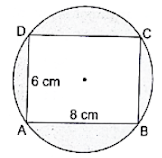A. 264 cm2

B. 266 cm2

C. 272 cm2

D. 254 cm2

Solution

Given

Length of rectangle = 8 cm

Breadth of rectangle = 6 cm

Area of rectangle = length × breadth

= 8 × 6

= 48 cm2

Consider △ABC,

By Pythagoras theorem,

AC2 = AB2 + BC2

= 82 + 62

= 64 + 36

= 100

AC √100 = 10 cm

⇒ Diameter of circle = 10 cm

Thus, radius of circle = 10/2 = 5 cm

Let the radius of circle be r = 5 cm

Then, Area of circle = πr2

= 22/7 × 5 × 5

= (22 × 25)/7

= 550/7

= 78.57 cm2

Area of shaded region = Area of circle – Area of rectangle

= 78.57 – 48

= 30.57 cm2

Hence, the area of shaded region is 30.57 cm2.

[None of the option is correct].

5. The circumference of a circle is 22 cm. Find its area. [Take π = 22/7]

Solution

Let the radius if circle be r

Circumference of circle = 22 cm

2πr = 22 cm

⇒ 2 × 22/7 × r = 22 cm

⇒ r = 22 × 1/2 × 7/22 cm

⇒ r = 3.5 cm

Area of Circle = πr2

= 22/7 × 3.5 × 3.5 cm2

= 38.5 cm2

∴ Area of Circle = 38.5 cm2

6. In a circle of radius 21 cm, an arc subtends an angle of 60° at the centre. Find the length of the arc.

Solution

Radius of circle = R = 21 cm

Angle subtended by arc = 60°

Length of an arc of a sector of angle θ° of a circle with radius R

= Circumference of circle × θ/360

= 2πRθ/360

Length of arc = 2 × 22/7 × 21 × θ/360 cm

= 22 cm

Length of arc = 22 cm

7. The minute hand of a clock is 12 cm long. Find the area swept by it in 35 minutes.

Solution

Length of the minute hand of a clock = 12 cm

∴ Radius = R = 12 cm

In 1 minute, minute hand sweeps 6°

So, in 35 minutes, minute hand will sweep 35 × 6° = 210°

Area swept by minute hand in 35 minutes = Area of a sector of angle θ° of a circle with radius R = πR2θ/360

= 22/7 × 12 × 12 × 60°/360°

= 264 cm2

Area swept by minute hand in 35 minutes = 264 cm2

8. The perimeter of a sector of a circle of radius 5.6 cm is 27.2 cm. Find the area of the sector.

Solution

Radius of circle = 5.6 cm

Perimeter of a sector of a circle = 2R + Circumference of circle × θ/360

= 2R + 2πRθ/360

Perimeter of a sector of a circle = 2 × 5.6 + 2 × 22/7 × 5.6 × θ/360 cm

= 27.2 cm

⇒ 2 × 22/7 × 5.6 × θ/360 = 27.2 – 11.2 cm

= 16 cm

⇒ θ = 16 × 1/2 × 1/5.6 × 7/22

⇒ θ = 163.63°

Area of sector = πr2 × θ/360 = 22/7 × 5.6 × 5.6 × 163.63/360

= 44.8 cm2

∴ Area of sector = 44.8 cm2

9. A chord of a circle of radius 14 cm makes right angle at the centre. Find the area of the sector.

SolutionChord AB subtends an angle of 90° at the centre of the circle

Radius of circle = R = 14 cm

Area of sector of circle of radius R = πR2θ/360

= 22/7 × 14 × 14 × 90/360 cm2

= 154 cm2

10. In the given figure, the sectors of two concentric circles of radii 7 cm and 3.5 cm are shown. Find the area of the shaded region.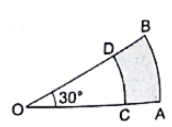Solution

Given,

Radius of smaller circle = R1 = 3.5 cm

Radius of bigger circle = R2 = 7 cm

Angle subtended = 30°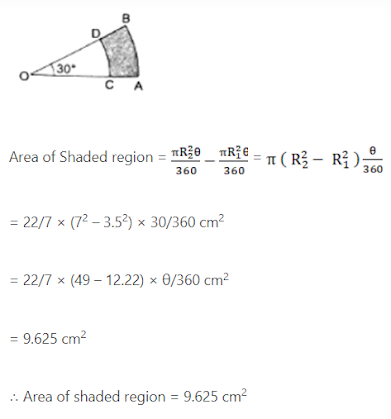11. A wire when bent in the form of an equilateral triangle encloses an area of 121√3 cm2. If the same wire is bent into form of a circle, what will be the area of the circle? [Take π = 22/7]

Solution

Let the sides of equilateral triangle be a cm

Area of equilateral triangle = 121√3 cm2

Area of equilateral triangle = √3/4 × a2

⇒ √3/4a2 = 121√3

⇒ a2 = 121√3 × 4√3 = 121 × 4 cm2

⇒ a2 = 484 cm2

⇒ a = 22 cm

Perimeter of equilateral triangle = 3a

= 3 × 22 cm

= 66 cm

Perimeter of equilateral triangle = Circumference of circle

Circumference of circle = 66 cm

Let the radius of circle be r

Circumference of circle = 2πr

⇒ 2πr = 66 cm

⇒ 2 × 22/7 × r = 66 cm

⇒ r = 66 × 1/2 × 7/22 cm

⇒ r = 10.5 cm

Area of circle = πr2 = 22/7 × 22/7 × 10.5 × 10.5 cm2

= 346.5 cm2

12. The wheel of a cart is making 5 revolutions per second. If the diameter of the wheel is 84 cm, find its speed in km per hour. [Take π = 22/7]

Solution

Diameter of the wheel = 84 cm

Let the radius of the wheel be R cm

Radius of the wheel = 84/2 cm = 42 cm

No. of revolutions wheel makes = 5 rev/sec

Since, 1 revolution = 2πR

Speed of the wheel = 5 × 2πR rev/sec

= 5 × 2 × 22/7 × 42

= 1320 cm/sec

= 13.20 m/sec

= 13.20 × 3600/1000 km/h = 47.52 km/h

Since, 1 m/sec = 3600/1000 km/h

13. OACB is a quadrant of a circle with centre O and its radius is 3.5 cm. If OD = 2 cm, find the area of (i) the quadrant OACB

(ii) the shaded region. [Take π = 22/7]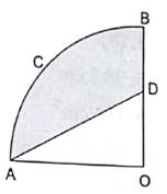Solution

Radius of circle = R = 3.5 cm

OD = 2 cm

OA = OB = R = 3.5 cm

Since, OACB is a quadrant of a circle

∴ Angle subtended by it at the centre = 90°

(i) Area of quadrant = πR2θ/360

= 22/7 × 3.5 × 3.5 × 90°/360° cm2

= 9.625 cm2

Area of triangle OAD = 1/2 × base × height

= 1/2 × OA × OD

= 1/2 × 3.5 × 2 cm2

= 3.5 cm2

Area of shaded region = 9.625 cm2 – 3.5 cm2

= 6.125 cm2

14. In the given figure, ABCD is a square each of whose side measures 28 cm. Find the area of the shaded region. [Take π = 22/7]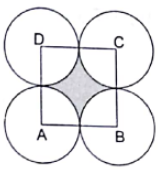Solution

Length of the sides of square = 28 cm

Area of square = a2 = 282 cm2

= 784 cm2

Since, all the circles are identical so, they have same radius

Let the radius of circle be R cm

From the figure 2R = 28 cm

From the figure 2R = 28 cm

R = 28/2 cm

⇒ R = 14 cm

Quadrant of a circle subtends 90° at the centre.

Area of quadrant of circle = πR2θ/360

= 22/7 × 14 × 14 × 90°/360° cm2

= 154 cm2

Area of 4 quadrants of circle = 154 × 4 cm2

= 616 cm2

Area of shaded region = Area of square – Area of 4 quadrants of circle

= 784 cm2 – 616 cm2

= 168 cm2

15. In the given figure, an equilateral triangle has been inscribed in a circle of radius 4 cm. Find the area of the shaded region. [Take π = 3.14 and √3 = 1.73]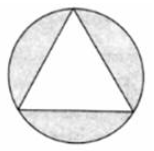Solution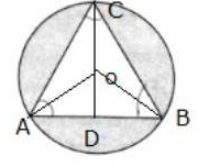Radius of circle = R = 4 cm

OD perpendicular to AB is drawn

△ABC is equilateral triangle,

∠A = ∠B = ∠C = 60°

OD/AO = sin 30°

AO = 4 cm

OD/AO = 1/2

⇒ OD = 1/2 × 4 cm

⇒ OD = 2 cm

= 42 – 22

= 16 – 4

= 12 cm2

= 2 × 2√3 cm

= 4√3 cm

Area of triangle ABC = √3/4 × AB2

= √3/4 × 4√3 × 4√3

= 20.71 cm2

Area of circle = πR2

= 3.14 × 4 × 4 cm2

= 50.24 cm2

Area of shaded region = 29.53 cm2

16. The minute hand of a clock is 7.5 cm long. Find the area of the face of the clock described by the minute hand in 56 minutes.

Solution

Length of minute hand = 7.5 cm

In a clock, length of minute hand = radius

Radius = R = 7.5 cm

In 1 minute, minute hand moves 6°

So, in 56 minutes, minute hand moves 56 × 6° = 360°

Area described by minute hand = πR2θ/360°

= 22/7 × 7.5 × 7.5 × 336°/360° cm2

= 165 cm2

17. A racetrack is in the form of a ring whose inner circumference is 352 m and outer circumference is 396 m. Find the width and the area of the track.

Solution

Inner circumference = 2πR1 = 352 m

⇒ 2 × 22/7 × R1 = 3652 m

⇒ R1 = 352 × 1/2 × 7/22

⇒ R1 = 56 m

Outer Circumference = 2πR2 = 396 m

⇒ 2 × 22/7 × R2 = 396 m

⇒ R2 = 396 × 1/2 × 7/22 m

⇒ R2 = 63 m

Width of the track = R2 – R1 = 63 m – 56m = 7 m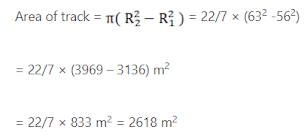18. A chord of a circle of radius 30 cm makes an angle of 60° at the centre of the circle. Find the areas of the minor and major segments. [Take π = 22/7 and 3 = 1.732.]

Solution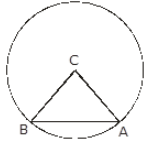∠ACB = 60°

Chord AB subtends an angle of 60° at the centre

In triangle ABC, AC = BC

So, ∠CAB = ∠CBA

∠ACB + CAB + ∠CBA = 180°

⇒ 60° + 2∠CAB = 180°

⇒ 2∠CAB = 180° – 60°= 120°

⇒ ∠CAB = 120°/2 = 60°

⇒ ∠CAB = ∠CBA = 60°

∴ △ABC is a equilateral triangle

Length of side of an equilateral triangle = radius of circle = 30 cm

Area of equilateral triangle = √3/4 × side2

= 1.732 × 30 × 30 cm2

= 389.7 cm2

Area of sector ACB = πR2θ/360

= 3.14 × 30 × 30 × 60°

= 471.45 cm2

Area of minor segment = Area of sector ACB – Area of △ABC

= 471.45 cm2 – 389.7 cm2

= 81.75 cm2

Area of circle = πR2 = 3.14 × 30 × 30 cm2

= 2828.57 cm2

Area of major segment = Area of circle – Area of minor segment

= 2826 cm2 – 81.75 cm2

= 2744.25 cm2

19. Four cows are tethered at the four corners of a square field of side 50 m such that each can graze the maximum unshared area. What area will be left ungrazed? [Take π = 3.14]

SolutionFrom the figure we see that cows are tethered at the corners of the square so while grazing they form four quadrants as shown in the figure.

Length of side of square = 50 m

Area of square = side2

= 502 m2

= 2500 m2

= 1/4 × 3.14 × 25 × 25 m2

= 490.625 m2

Area of 4 quadrants = 4 × 490.625 m2

= 1962.5 m2

Area left ungrazed = Area of shaded part

= Area of square – Area of 4 quadrants

= 2500 m2 – 1962.5 m2

= 537.5 m2

20. A square tank has an area of 1600 m2. There are four semi-circular plots around it. Find the cost of turfing the plots at Rs 12.50 per m2. [Take π = 3.14]

Solution

Let the length of side of the square tank be a

Area of square tank = a2 = 1600 m2

⇒ a = √1600 m

= 40 m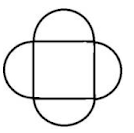Let the radius of semicircle be R

From the figure we can see that

Length of the side of the square = Diameter of semicircle

40 m = 2 × R

⇒ R = 40/2 m

⇒ R = 20 m

Area of semi-circle = 1/2 πR2

= 1/2 × 3.14 × 20 × 20 m2

= 628 m2

Area of 4 semi-circles = 4 × 628 m2

= 2512 m2

Cost of turfing the plots = Rs 12.50 per m2

Cost of turfing = Cost of turfing per m2 × Area of 4 semicircle

= Rs 12.50 × 2512

= Rs 31400